Enter your search above to see Results

Whitepapers

# The Killer Issue When Computing Fuzzy Matching

A whitepaper to discuss compute problems when fuzzy matching.

### Introduction

A very common task in business is computing a probabilistic match between two strings. This is the so called fuzzy matching algorithm. Common algorithms in this group measure just how similar the two text strings are. This is important because we might have two strings which are technically different but which may refer to the same thing. For example, we might have an innocent spelling mistake in our data. In our example the company Eclipse Systems has been entered correctly to the list but has also been entered as Eclispe Systems. Clearly the strings are different, but there is a high probability that they mean the same company.

### Removing Duplicates

Many companies have problems when the start to use probabilistic matching and, in this paper, we would like to highlight just one of the issues that one faces. The scenario that we will consider is removing duplicates from a list. We have five companies in our list:

– Eclipse Systems
– Cornerstone Data
– Eclispe Systems
– Peach Computers

The best way to search for duplicates is to compare each string with each other in a grid and to measure the distance with a common algorithm. Here is the grid filled in with the cosine distance between the strings.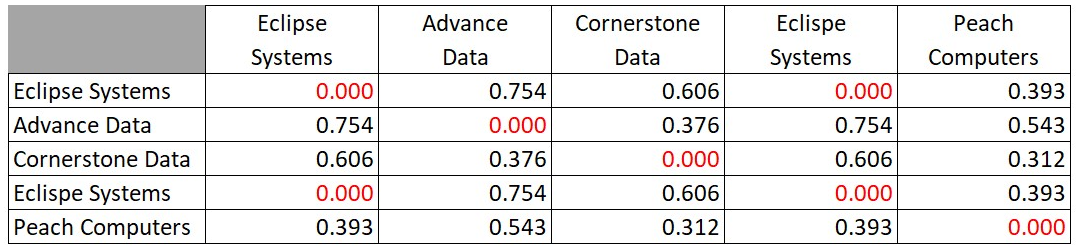You will notice that the algorithm shows a distance of zero between Eclipse and Eclispe and these could be considered to be duplicates. So, this small example is very clear and very easy to do. Although we showed the full matrix above, we obviously don’t need to compute the values on the diagonal because they have to be zero by definition. We also notice that we have every distance written twice. This is because the distance from A to B must be, by definition, the same as the distance from B to A. The only calculations that we need to do are either the half of the grid above or below the diagonal, like this: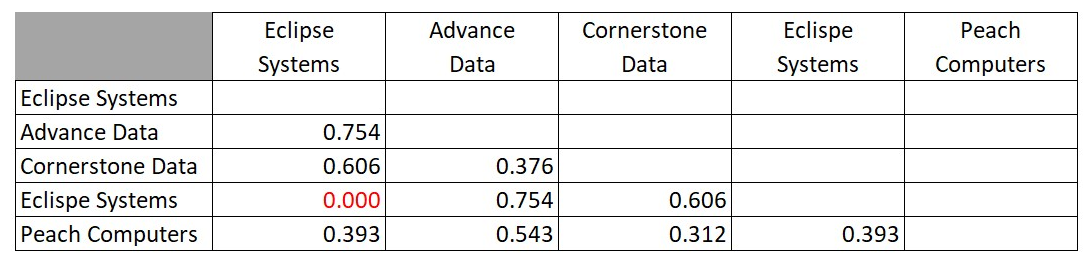This is a total of ten numbers because the five by five grid has twenty-five squares, but the diagonal has five. This leaves a total of twenty squares above and below the diagonal, but we only need one side of the diagonal. So, we pick half which gives us ten calculations. If $n$ is the size of our list, then the number of calculations is $N$ where:

$N = \frac {n^2-n} {2}$

Now we come to our issue of why there are compute problems when fuzzy matching. The equation for $N$ has an exponential term. It is a quadratic equation and so the chart of $N$ against $n$ will be a parabola. Unfortunately, in all math texts parabolas are not well represented. They always seem to look like this: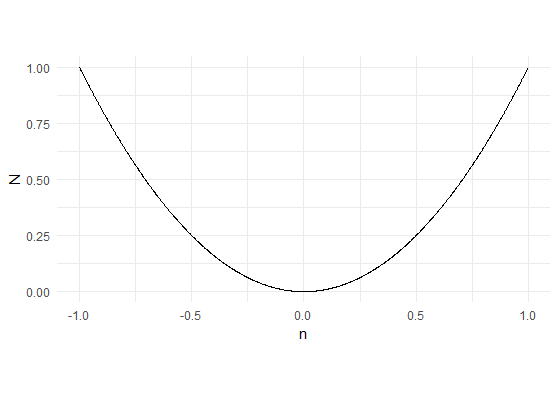This is perfectly correct; however, it shows just the detail of what happens near to zero. We get the impression that the curve is quite flat and open, but this is very far from the truth. Here is the same chart but showing the values n from -15 to +15 and keeping the x and y scales the same.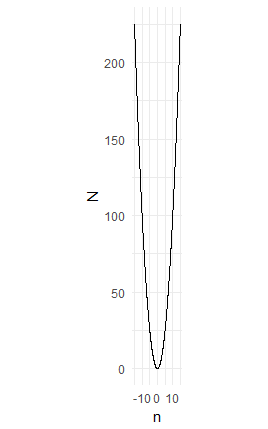Suddenly we see the parabola in its true light. It is, in fact, thin, sharp and steep and, more to the point, it keeps getting steeper as it gets higher. However, this was for still very small values of 15. Here is the chart for 200: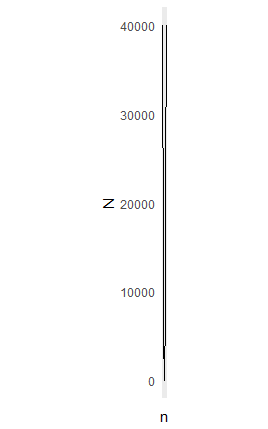### Wheat on the Chessboard

This issue has been well known for a long time. There is a story which dates from 1256 about someone asking for a quantity of wheat. The quantity was to be defined on a chessboard where the first square has one grain, the second square two grains, the third square four grains and so on. Each square has double the quantity of the previous square.

When someone imagines this quantity, we can easily visualize the first four of five squares and it all looks very benign. We are seeing the open and flat parabola and not the sharp one. The total number of grains on the first half of the chessboard is 4,294,967,295 which equates to 279 tons of wheat. Clearly an amount that is possible to deliver.

Unfortunately, on the entire chessboard there would be 18,446,744,073,709,551,615 grains of wheat, weighing about 1,199,000,000,000 tons. This is many thousands of times greater than the world’s entire production. The number is so big that it is hard to imagine. If it were a distance in meters, then it would be equivalent to 2,000 light years. Yet, in just one second, light goes around the Earth more than seven times.

You can read more at the Wikipedia article on the chessboard https://en.wikipedia.org/wiki/Wheat_and_chessboard_problem

### The computational issue with fuzzy matching

The matrix that we showed above took around 2 milliseconds to compute each value in the grid. Let us then estimate how long it will take to find duplicates in different sizes of list. The processing time $T$ can be estimated thus.

$T \approx \frac {n^2-n}{2} \times \frac{2}{1000}$ Seconds

$T \approx \frac {n^2-n}{2} \times \frac{2}{1000} \times \frac{1}{60.60.24}$ Days

• A list of 100 has T of 10 seconds. Great.
• A list of 1,000 has a T of 15 minutes. Reasonable.
• A list of 10,000 has a T of just over a day. Unacceptable.
• A list of 100,000 has a T of nearly four months.

This is that very steep and sharp parabola in action. Unfortunately an N of 100,000 is entirely possible in business. It is not unusual for a large company to have this many customers.

### The wrong solution

So often we see people attempting these calculations on parallel processors or networks of computers. This is a mistake for the simple reason that this is a linear solution to an exponential problem. Having ten computers will reduce the time by a factor of 10 but it would be much better if the factor were $10^2$. It is for this reason that parallel processing seems to be a solution for certain sizes. A cluster of 10 processors would make the list of 10,000 a reasonable proposition needing only a few hours to run. The list of 100,000, however still needs nearly twelve days to process. We are not saying that it is wrong to use parallel processing when text matching but there are better techniques.

### The Silver Bullet

It is, perhaps, obvious that if increasing the list size causes an exponential problem then reducing the list size causes an exponential solution. Any reduction in the size of the list causes an exponential improvement in compute time. Imagine if we could take our list and create clusters of names where we think it is highly likely that the duplicates are to be found. Suppose we can separate our list into $m$ such clusters of equal size. We then run our algorithm for each of the $m$ clusters. The processing time is the total of the time to complete cluster 1, cluster 2, until cluster $m$.

$T \approx \frac{2}{1000} \sum_1^m \frac {(n/m)^2-(n/m)}{2}$ Seconds

This is what this means in practice.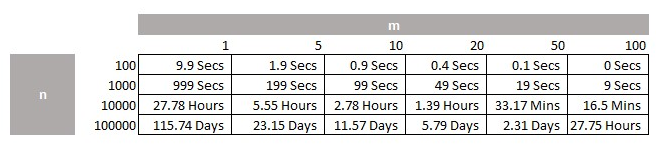We can see that even our largest list of 100,000 becomes practical to process as long as we can get at least twenty clusters.  This is one solution to compute problems when fuzzy matching.

There is a downside to the technique. Obviously, if a member of cluster 1 has a duplicate in cluster 2 then the process will not detect this so our approach could be imperfect. Fortunately, we have two answers to this problem.

Firstly, the key is to discover clusters where there is a very high likelihood of having a concentration of duplicates. Therefore, it pays to employ the expertise of a data scientist with the mathematical skills to be able to do this effectively. Secondly, once we have removed duplicates our list becomes smaller and we can rerun against a smaller list.

This technique is a quadratic solution to a quadratic problem. Parallel processing is a linear solution to a quadratic problem.

### The Gold Standard When Fuzzy Matching

At JTA we have many techniques to preprocess lists of texts in a way that removes differences in interpretation in a company name. This means that, after we have run the process, we can find a great many duplicates or matches which are exactly equal. In other words, we don’t need to use probabilistic matching. We can just run a process to find exact repetitions in the list. This is a linear process and very fast to do, even in a large list. We only run the probabilistic match on the remaining items after we have found the direct match. We have found that we can reduce the size of the probabilistic problem by 75% before we run it. This significantly reduces the compute problem when fuzzy matching.  To give you an idea of the impact here is the above table of processing times when we preprocess.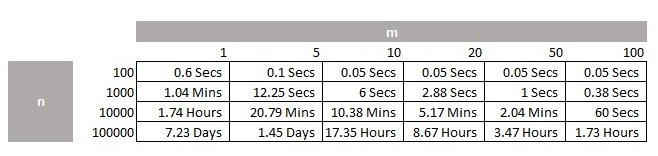### Conclusion

• We started with a mathematical explanation as to why there are compute problems when fuzzy matching.
• Later we saw how this would create a business problem that took nearly four months to process.
• Then we learned that there are techniques that offer a solution that is very nearly as good but which takes 28 hours.
• Then we learned that techniques can be combined to give a solution that takes less than two hours to run.
• None of the solutions require large computer clusters or parallel processing.
Enquiry